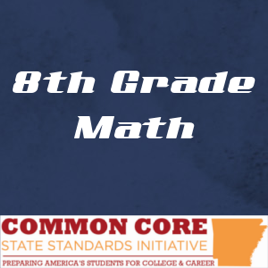21 episodes

This collection contains lessons created by a variety of Arkansas teachers that address specific Common Core State Standards. They were compiled and produced by the NEA Portal.# Grade 8 Mathematics for Common Core State Standards Arkansas

• Education

This collection contains lessons created by a variety of Arkansas teachers that address specific Common Core State Standards. They were compiled and produced by the NEA Portal.

• video
Algebraic Expressions

## Algebraic Expressions

CC.8.NS.2 – Use rational approximations of irrational numbers to compare the size of irrational numbers, locate them approximately on a number line diagram, and estimate the value of expressions (e.g., π2).

• 5 min
• video

CC.8.NS.2 – Use rational approximations of irrational numbers to compare the size of irrational numbers, locate them approximately on a number line diagram, and estimate the value of expressions (e.g., π2).

• 8 min
• video
Laws of Exponents 1

## Laws of Exponents 1

CC.8.EE.1 – Know and apply the properties of integer exponents to generate equivalent numerical expressions.

• 8 min
• video
Laws of Exponents 2

## Laws of Exponents 2

CC.8.EE.1 – Know and apply the properties of integer exponents to generate equivalent numerical expressions.

• 6 min
• video
Law of Exponents 3

## Law of Exponents 3

CC.8.EE.1 – Know and apply the properties of integer exponents to generate equivalent numerical expressions.

• 10 min
• video
Multiplying Monomials

## Multiplying Monomials

CC.8.EE.1 – Know and apply the properties of integer exponents to generate equivalent numerical expressions.

• 4 min